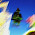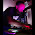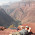## Saturday, 5 November 2011

### Lost in Maths! (2D Sound location with 4 sensors)

This post is about my ongoing project to do fast 2D sound location on a ping pong table, this is some ideas I've been having and wanted to share - since I need some help!

Ok here's the problem... we have a rectangular area ABCD and somewhere in that area (point X) there is an event that produces a sound (e.g. a ping pong ball strikes the surface). This results in sound waves travelling outwards to the corners where they are picked up by sound detectors.

The first sound to be picked up at each sensor arrives at a time that obviously depends on that sensor's distance from the original sound. Lets call the times tA, tB, tC, tD. (Each sensor will pick up reverberations and echoes after the original sound, but its the first "edge" of the sound we're interested in)

Now since we don't know when the original sound happens (we only detect it when it got to the closest sensor) we don't actually know the real values of tA, tB, tC, tD but we get relative times from the first sensor. In this case X is closest to D,  the sound reached D first. The time we read at each sensor A,B,C is relative to tD. Graphically this can be shown like this (each red line is reduced by distance XD)

Now we can use these reduced distances to define circles based on the points A,B,C and D (radius at D is initially zero)

As pointer out by Arduino forum member Necromancer on http://arduino.cc/forum/index.php?topic=52583.0 we can find X  geometrically by progressively increasing the radii of the circles at A,B,C and D by the same amounts until all the circles intersect at a single point. At this point we have added back the unknown distance XD and the circles intersect at point X as shown below.

The problem is that this iterative calculation of circle intersections is processing-heavy and would probably take too long to solve on a microcontroller to be responsive enough for my application. However I started thinking about getting a "head start" by doing some simple calculation to get the initial increment where all the circles intersect for the first time, and go forward from there.

Here's what I mean.... point Q is a point on the line AC which is equal distances from the points where the initial circles around A and C cross AC.

The actual coordinates of Q aren't needed, we just need to know how much to increase the radii of the circles around A and C so that they intersect at the first time (which will happen at Q)

The calculation is simply the length of AC minus the radii of the two circles, all divided by two. If we calculate this value and add it to the radii of the circles they will meet at Q.

If we do the same calculation for lines AB, BC, CD, AD, AC, BD we'll get 6 different values that we should increase circle radii by make them intersect. We can just take the largest of all the 6 values and apply that as the base value by which the radii of all the circles should be increased to get to the initial point where all the circles intersect.

This will not neccessarily be  point X... we might need to interatively expand the circles a bit more to make them all intersect at the same point. However we got a big head start and so far we've just done simple arithmetic.

I made a simple program where I could click with the mouse to simulate the values arriving at the 4 sensors., then applied the above calculations... in many cases the results are very close to the final point X. For example:

In other places the accuracy is not so spot-on, but it looks like some kind of simple averaging of the points of intersection might give a position good enough for what I want, and without having to iteratively apply complex calculations (like square roots), so it should be pretty fast.

Only three sensors are strictly required for multilateration, however I found that adding the fourth sensor made a massive difference to the accuracy of the above calculation.

The next step is to calculate the points of intersection and give it a try with some averaging, to see if I can avoid the iterative method. However I'm not a mathemetician, so if anyone has a better idea, please let me know!

Update: OK, I gave the averaging thing a try. This clip shows my test program using these calculations to track the mouse pointer. The tracking is not perfect and there are a couple of places (vertical midpoint of the area, towards left and right side) where it is worst, but I think this is good enough (especially given that my sensors won't be perfect!).

The program displays the fours circles calculated as above. The final calculated position is displayed by the red crosshairs

The process is as follows:

1. Firstly I need to simulate the sensor inputs, so I take the position of the mouse pointer, calculate distance to each corner and then subtract the minimum distance from all the others. The results (tA, tB, tC, tD) are representative of time or arrival info from real sensors.

2. For each edge, and the two diagonals I get the length of the line (area width, height or diagonal distance) and subtract the relative arrival times for the points at each end.

offset1 = (width - tA - tB)/2
offset2 = (width - tC - tD)/2
offset3 = (height - tA - tD)/2
offset4 = (height - tB - tC)/2
offset5 = (diagonal - tA - tC)/2
offset6 = (diagonal - tB - tD)/2

Now take the maximum of these 6 values:

offset = max(offset1,offset2,offset3,offset4,offset5,offset6)

now calculate the circle radius by adding the offset to each time.

rA = tA + offset
rB = tB + offset
rC = tC + offset
rD = tD + offset

now calculate the intersection points of all pairs of circles. I used the C code example from here  http://paulbourke.net/geometry/2circle/

There are six pairs of circles, AB, AC, AD, BC, BD, CD. Not all may intersect (ignore pairs which do not intersect). Otherwise we get 2 insection points (which may be identical if circles just touch) for each pair of circles. Lets say that for each pair of circles we can get two intersection points P and P'

For each pair of points P and P', one will be closest to our target point and the other should be discarded. The way I did this was to calculate the average of all the points, then go back through the list selecting the point from each pair that was closest to the calculated average point and then averaging just these "closest points".

ie. Take the first "rough" average of all points P and P' - lets call is (Xave, Yave), then recalculate the average position using either P or P' from each pair based on the condition:

if (Xp - Xave)^2 + (Yp - Yave)^2  > (Xp' - Xave)^2 + (Yp' - Yave)^2
then use point P'
else use point P

The resulting average (X , Y) is the final calculated point.

Better accuracy would be got by interatively increasing rA, rB, rC and rD and recalculating the intersection points until they are at their closest to each other. However I don't think I need this - the sensor input is unlikely to be so accurate it would benefit from this.... and I think it would be computationally expensive due to calling sqrt( ) many times and therefore slow.

Once again I'm no mathematician and I'd be grateful for any advice here!

1.Just off the top of my head (so it may not even be feasible) but what about using pythagoras and some simple y=mx+c type line equations on two of your lines.

Calculate the gradient (m) for line1 and line2 using simple trig (sin/cos) and where the two lines intersect (i.e. the equations y=mx+c are equal for both) you'd have your X and Y values. I did similar stuff for a Flash-based game a few years ago and it worked quite well. You might need floating point maths on your mcu to do it though.

2.Just another thought - http://www.teacherschoice.com.au/maths_library/trigonometry/solve_trig_sss.htm shows how to solve triangles. Using the AC and DB diagonals, and given you know AB, BC, CD and DA, you could solve all this with triangles.

But on a microcontroller, you tend to use look-up tables for sin/cos operations anyway to speed things up. So why not create an app which splits the table top up into a grid of fine enough resolution, pre-calculate all the values for X at each point, shove them all in a big look up table, then compare when you get the signals in?

How fine do you need your resolution? Would a grid of say 20 x 10 be accurate enough? Just get a PC to do all the crunchy calculations up front, and do simple comparisons on the mcu at runtime?

3.I'm working on a similar problem for school (an electronic target) and have some suggestions.

1. I'm not really sure how ping pong tables are constructed, but you might run into problems with the propagation speed differing depending on direction. Wood propagates sound significantly faster along the grain that across it. I imagine the table is made of plywood, so it might even out since the individual plies alternate. MDF would probably be okay. Obviously, a solid plastic or aluminum table would be best.

2. If you're triggering event is just a basic threshold, your triggering will be increasingly off as the source moves closer to one sensor than the other since one sensor will receive a significantly greater signal and will trigger much earlier than the other. To get around this, we use a combination of threshold triggering and zero detection. We time based on the first zero crossing after a threshold is reached. Depending on the accuracy you need, though, threshold triggering might be fine.

3. In order to solve the location from the TDOA numbers, you have to calculate some hyperbolas (you can also do it experimentally as you've seen but that's very slow). Every pair of sensors will give you one valid hyperbola and the intersection of two hyperbolas will give you the source location. All you need is, for each pair, which triggered first, the time difference, and the propagation speed. You can do this with three sensors, but you'll need to know the propagation speed.

4. In order to figure out the propagation speed, we use four sensors to build four hyperbolas (you could do six but we haven't figure out how to do the diagonal ones yet). This will give you several possible source points and then you can adjust the propagation speed until they all line up (as closely as possible). You can estimate error by calculating the standard deviation of the closest you can get.

You're probably wondering how to solve the hyperbolas. I have spent a ridiculous amount of time trying to figure that out, reading through journal articles and theses that were of no help. This guy teaches a class on it and breaks it down in a very useful way:

http://www.ee.nmt.edu/~rison/ee389_spr08/

I made very little progress solving the hyperbolas until I found this. Particularly, look at (1) on "algebra to show..." Nice and easy to solve for x and y.

4.Thanks for your help guys! Interesting point about the zero crossing, Matt. I'd not thought about that... I hope I can set the sensitivity on the sensors close enough to be "good enough" for what I need. Now to digest all this great info.
Cheers!

5.I've been thinking about this problem since you told me about it a couple of weeks ago. One thing that strikes me as being a slight spanner in the works is that the majority of table tennis tables are hinged in the center for easy storage. If this is the case you will not be able to use the method the way you have suggested since the vibration through the table will stop dead at the hinge, or if it should transit it will likely ruin your timings. The only solution i can see to resolve this would be to apply the above method on each side (either 8 of 6 sensors in total).
How rude of me, it's Antony by the way, i came to visit BB a couple of weeks ago (interested in Midi!).

6.E-mail me sometime (click the contact link on my site) and I'll send you some stuff you might find useful.

7.Two words: Sound ranging

8.9.This comment has been removed by the author.

10.Okay, I removed my previous comment because it didn't jive well with me.
Basically the first sensor to hear a sound triggers a timer. Then the difference between the first sensor and the other sensors hearing a sound will be used to calculate the radii of the sound relative to the first timer that hears it.
Use that with some trig to find the location of the ball.
Is it that simple? I don't know but I figured I'd throw it out there.

11.Anyway you could post your code?

12.Sure- you can see the code here
https://github.com/hotchk155/NoisyTableSensing/blob/master/NoisyTableSensing.ino

13.Awesome blog! Just catching up. I see you did this over a year ago! I think the math can be SIGNIFICANTLY easier if you use the fact that tA^2*tC^2 = tB^2*tD^2. You have to use the unknown tI of the time between original impact and the first sensor firing and deal with your propogation speed in order to actually 'solve' the triangle but the fact that you sensors are known distances apart and at known angles allows you to assume an unknown constant for velocity in the law of cosines and you can solve that twice for a two adjacent angles at a corner to get all your unknowns.

I REALLY like that circles video that you posted above. Is that SW somewhere I can download? I'd like to try it with some additional calculations for my system!

1.sorry, just reread my post...the equation is tA^2+tC^2 = tB^2+tD^2.

And if the tI comment doesn't make any sense, what I mean is that one of your lines from a sensor to the impact will have tX of 0 and that distance is actually tI*speed of propogation (in your example we're talking about tD) and then tA is tI plus some actual time DIFFERENCE of arrival from sensor D to sensor A.

14.Hey Jay
very interested in your comment.. I never did find a better mathematical solution but decided the accuracy of my maths approach was appropriate to the (rough) precision of the sensing. We moved forward with that and "noisy table" went out in public last year
http://vimeo.com/45745840
http://www.fact.co.uk/projects/noisy-table/
drop me an email jason_hotchkiss at hotmail dot com if you want to talk maths :) and thanks for your comment

15.http://pppp.media.mit.edu/

A ping pong table that sensed ball hit locations and displayed projected visualizations based on the hits.

1.wow! thats spooky... very similar. They also have some very interesting differences in sensor locations and signal conditioning electronics that I need to try to understand! Thanks for the link

16.Accuracy is 1-2 inch which is really good, but I can't see any schematic how they connect it to Arduino nor I see any code. Maybe not an open source project. What about the accuracy of your ball recognition?